# How do you calculate valency of radicals? Are there any easy

How do you calculate valency of radicals? Are there any easy ways to remember the valency for radicals? What is the valency of transition metals?

Here are some examples to work on valency of radicals:
Framing of Formulae of compounds with the Help of Valency

A compound is a substance formed by the combination of atoms of two or more elements. To be able to frame formulae, one should be thorough with the valencies.

1. Frame the formula of the compound formed when sodium and chlorine combine.
Write down the symbol of sodium and chlorine and draw a short line next to each as shown. The short lines represent the valency (just the number and not the charge) of each atom.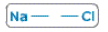Connect each valency, with the valency of the other.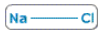Now there are no free valencies. So the formula is NaCl.
2. Frame the formula of the compound formed when calcium and chlorine combine.
Write down the symbol of calcium and chlorine, and draw 2 small lines next to calcium (valency=
3. and 1 small the next to chlorine.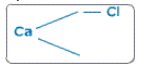Join the valencies of calcium with that of chlorine.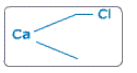Here one valency of calcium is free. So take one more chlorine.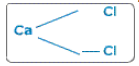Join them.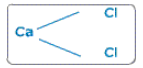So we get the formula CaCl2.
More Examples: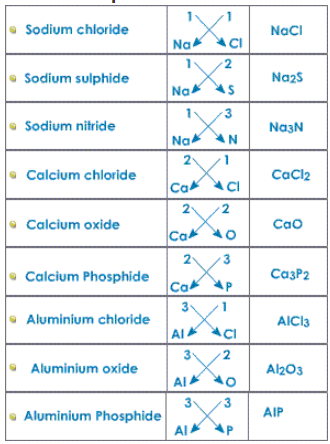The valency for transition metals is variable.